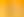Bringing Life to Data

# Stages1. Class Statistics

EXPLORE

Suggested Learning Intentions

• To gather and organise data to answer questions about our world
• To explore various graphic techniques and explain how they provide a different picture of the shape of the data

Sample Success Criteria

• I can gather and organise my data to answer questions about the sample population the data came from
• I can demonstrate my solution and thinking using a variety of manipulatives
• I can describe key features of graphs
• I can construct graphs in ways that help me represent and understand data
EXPLORE

2. Talking Rubbish

EXPLORE

Suggested Learning Intentions

• To design questions that can be answered using statistics
• To investigate how data can be used to solve real world problems
• To describe data using numbers; these are called statistics

Sample Success Criteria

• I can design a question to investigate that can be answered using statistics
• I can collect, analyse, and interpret data to address a real-world problem
• I can model data using a variety of manipulatives
• I can describe data using statistics
EXPLORE

3. Demographic Diversity

EXPLORE

Suggested Learning Intentions

• I can design a question to investigate that can be answered using statistics
• I can collect, analyse, and interpret data to address a real-world problem
• I can describe data using statistics

Sample Success Criteria

• I can design a question to investigate that can be answered using statistics
• I can collect, analyse, and interpret data to address a real-world problem
• I can describe data using statistics
• I can model data using a variety of manipulatives and use this to analyse the data
EXPLORE

4. Welcome to the Global Village

EXPLORE

Suggested Learning Intentions

• I can design a question to investigate that can be answered using statistics
• I can collect, analyse, and interpret data to address a real-world problem
• I can describe data using statistics

Sample Success Criteria

• I can explain how populations differ using statistics and graphic techniques
• I can model data using manipulatives
• I can justify my choices of how I represent data visually or with statistics
• I can communicate my findings effectively
EXPLORE